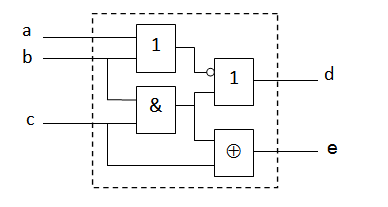Warning

# 1. Seminar - Hardware description language - Introduction to Verilog

Icarus Verilog will be used for digital logic designs.

On Debian/Ubuntu GNU/Linux system you install required software from commadline by

sudo apt install iverilog gtkwave

GTKWave (http://gtkwave.sourceforge.net/) utility is used to visualize simulated signals timing diagrams.

QtMips (https://github.com/cvut/QtMips/) will be used as a CPU model for work on seminaries. Refresh your knowledge of basic CPU architectures. See B35APO Computer Architectures course.

Alternatives:

The course goals:

• Understand modern CPU and GPU architectures to use them in full power
• Ability to join development of new CPU design or use it in custom solutions (i.e. RISC-V)
• Gaining experience by implementation of simple single cycle and then pipelined CPU (MIPS compatible QtMips)
• Basic how to utilize processing parallelism on all computational systems levels

Short introduction to Verilog: English PDF: verilog-en.pdf ODP: verilog-en.odp YouTube: Czech 2021 Winter

Git repository with verilog templates: https://gitlab.fel.cvut.cz/b4m35pap/stud-support

#### Exercise 1.

Consider the combinatorial circuit shown in figure below. Describe it in Verilog.Solution. We will create a new file my_circuit.v with the following content:

module my_circuit(input a, b, c,
output d, e);

assign d = ~(a | b) | (b & c);
assign e = (b & c) ^ c;

endmodule

#### Exercise 2.

Create the TestBench for the simulation of the combinatorial circuit from Exercise 1; and perform the simulation.

Solution. We will create a new file, e.g. my_circuit_tb.v, which defines inputs to the circuit (stimulus list). Then we are able to simulate the circuit and observe its outputs.

module test();
reg a, b, c;
wire x, y;

my_circuit my_circuit_XY(a, b, c, x, y);

initial begin
$dumpfile("test.vcd");$dumpvars;
a=0;
b=0;
c=0;
#160 $finish; end always #20 a = ~a; always #40 b = ~b; always #80 c = ~c; always @(x)$display( "The value of x was changed. Time=%d, x=%b. Inputs: a=%b, b=%b, c=%b.",\$time, x,a,b,c);

endmodule

In the command line we will compile both the files, run the simulation, and visualize the results by following commands:

iverilog my_circuit.v my_circuit_tb.v
./a.out
gtkwave test.vcd

Alternatively, we can use vvp instead of gtkwave:

iverilog -otest.vvp my_circuit.v my_circuit_tb.v
vvp test.vvp

#### Exercise 3.

Sketch (by the hand) a schematic of the circuit according the following description:

  if A is equal to 1,
then Y is equal to (B and C)
else Y is equal to (B xor D) or C
The variables A, B, C and D are logical variables.

#### Exercise 4.

Describe the logic circuit from Exercise 3 in Verilog and perform the simulation. What is the value of Y in the case when A==0, B==1, C==0, D==0? Support your statement by the simulation results.

#### Exercise 5.

Describe in Verilog following circuit. Keep in mind that it is composed from three identical circuits according the Exercise 1. Use structural description. What is the output when A==0, B==1 and C==1?